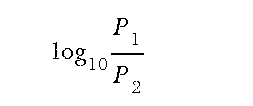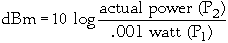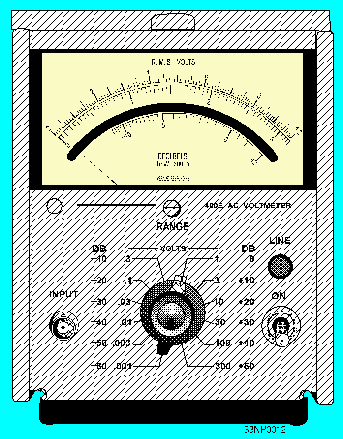tachometer, stroboscope, and the strobotac. Explain the measurement of frequency in various ranges using vibrating reeds, tuned circuits, heterodyne frequency meters, absorption wavemeters, cavity wavemeters, and frequency counters. Describe the use of frequency-measurement devices, oscilloscopes, and spectrum analyzers in waveform analysis and maintenance. Describe semiconductor testing and applicable terms in maintenance. "> Miscellaneous MeasurementsCustom SearchMISCELLANEOUS MEASUREMENTS

LEARNING OBJECTIVES

Upon completing this chapter, you should be able to:

• Define and explain the use of the terms "dB" and "dBm" as they apply to power measurements.
• Describe the use of resistive loads, bolometers, and thermocouples in power measurements.
• Explain the measurement of mechanical rotation using the tachometer, stroboscope, and the strobotac.
• Explain the measurement of frequency in various ranges using vibrating reeds, tuned circuits, heterodyne frequency meters, absorption wavemeters, cavity wavemeters, and frequency counters.
• Describe the use of frequency-measurement devices, oscilloscopes, and spectrum analyzers in waveform analysis and maintenance.
• Describe semiconductor testing and applicable terms in maintenance.

In chapter 1, you studied test equipment administration and the basic measurements that all technicians are responsible for performing. Topic 2 presents miscellaneous measurements that are fairly common; keep in mind, however, that you may not routinely perform these measurements in your particular job. This chapter introduces you to several test instruments and components found in those test instruments. It will also serve as a review of some of the basics of electronic theory related to test equipment.

POWER MEASUREMENTS

You may be required to check the power consumption and the input-signal power levels of electronic equipment. The determination of dc power is fairly simple; recall that the unit of power, the watt, is the product of the potential in volts and the current in amperes (P = E X I).

As discussed in NEETS, Module 2, Introduction to Alternating Current and Transformers, the phase angle of the voltage and current must be considered for accurate ac power measurements. The measurement of ac power is further complicated by the frequency limitations of various power meters. If there is no phase angle difference, you can compute ac power in the same manner as dc power; that is, by determining the effective value of the product of the voltage and current.

For equipments that operate in the audio-frequency (af) range, power levels have to be determined in the performance of routine checks and during corrective maintenance procedures.

Power measurements for af circuits are usually indicated in terms of decibels (dB) or decibels referenced to 1 milliwatt (dBm). Because the actual calculation of decibel measurements is seldom required, the following explanation is somewhat simplified. Most test equipment is designed to measure and indicate decibels directly. This eliminates the need for you to perform complicated calculations. Nevertheless, a basic explanation of the decibel measurement system is necessary for you to understand the significance of dB readings and amplifier-gain ratings that are expressed in decibels.

THE DECIBEL SYSTEM

The basic unit of measurement in the system is not the decibel; it is the bel. The bel is a unit that expresses the logarithmic ratio between the input and the output of any given component, circuit, or system. It may be expressed in terms of voltage, current, or power. Most often, it is used to show the ratio between input and output power to figure gain. You can express the power gain of the amplifier (N) in bels by dividing the output (P1) by the input (P2) and taking the base 10 logarithm of the resulting quotient. The formula for determining this gain is:If an amplifier doubles the input power, the quotient of P1to P2 will be 2. If you consult a logarithm table, you will find that the base 10 logarithm of 2 is 0.3, making the power gain of the amplifier 0.3 bel.

Q.1 What is the logarithmic ratio between the input and output of a given circuit called?Experience has shown that because the bel is a rather large unit, it is difficult to apply. A more practical unit, and one that can be used more easily, is the decibel (1/10 bel). You can convert any figure expressed in bels to decibels by multiplying that figure by 10 or simply by moving the decimal point one place to the right. Applying this rule, we find that the above ratio of 0.3 bel is equal to 3 decibels.

The decibel (dB) cannot be used to represent actual power; only the ratio of one power compared to another. To say that an amplifier has a 3 dB gain means that the output power is twice the input power. This gives no indication of the actual power represented. You must be able to state the input power for it to be meaningful. In many applications, a mathematical expression represents the actual power, not a power ratio. One standard reference is the dBm.

The dBm is an abbreviation used to represent power levels above or below 1 milliwatt. Negative dBm (-dBm) represents power levels below 1 milliwatt, and positive dBm (+dBm) represents power levels above 1 milliwatt. In other words, a dBm value is a specific amount of power; 0 dBm is equal to 1 milliwatt. Briefly stated, the amount of power in a given value of dBm is the power which results if 1 milliwatt is amplified or attenuated by that dB value. For example, 40 dBm represents an actual power level (watts or milliwatts) that is 40 dB above 1 milliwatt, whereas -10 dBm represents a power level that is 10 dB below 1 milliwatt. The formula for finding dBm is a variation of the dB power formula:Q.2 What term is used to represent power levels above or below a 1-milliwatt reference?You do not need to use the formula in most applications. The following shows conversions of dBm to mW:

 +20dBm=100mW +10dBm=10mW +7dBm=5mW +6dBm = 4mW +4dBm=2.5mW +3dBm=2mW 0dBm=1mW -3dBm=.5mW -10dBm=.1mW

For a +10 dBm level, start with the 1 milliwatt reference and move the decimal point one place to the right (+10 dBm = 10 mW). Another 10 dB increment brings the power level to +20 dBm, thereby moving the decimal point another place to the right (+20 dBm = 100 mW). For a -10 dBm level, again start with 1 milliwatt, but this time move the decimal point one place to the left (-10 dBm = .1 mW). An additional 10 dB decrease results in another decimal point shift to the left (-20 dBm = .01 mW).

For a 3 dB increase, you double the power. For a 3 dB decrease, you reduce the power by one-half (+3 dBm = 2 mW and -3 dBm = .5 mW). A +6 dBm level is an additional 3 dB change from +3 dBm. In this case, you just double the power level of the +3 dBm (+6 dBm = 4 mW).

Q.3 What milliwatt value is equal to +6 dBm?The dB change can be made in either direction. For example, +7 dBm is a decrease from +10 dBm. Reducing the +10 dBm power by one-half, we have +7 dBm, or 5 mW. A +4 dBm power level is a 3 dB decrease from +7 dBm (+4 dBm - 2.5 mW). By using this simple method, you can quickly find any power level that corresponds to a given dBm.

Some test instruments you will be using are calibrated in decibels and have a 1 milliwatt zero reference level. Figure 2-1 illustrates such an instrument. Notice that this is an ac voltmeter in which the upper scale of the meter indicates ac voltage and the lower scale indicates decibels. The zero power-level indicator on the decibel scale is located at, or near, center scale. If the power in the line being measured is more than the reference value, the meter will indicate a value to the right of the zero mark (+dB). If the power is less than the reference value, the meter will indicate a value to the left of the zero mark (-dB). Such meters are useful when recording measurements where a direct indication in decibels is desired. However, you must remember that this meter is still a voltmeter and that power measurements are not meaningful unless the circuit impedance is known. If you feel the need to review how to calculate power in ac circuits, refer to NEETS, Module 2.

Figure 2-1. - Ac voltmeter.At radio frequencies below the UHF range, power is usually determined by voltage, current, and impedance measurements. One common method used to determine the output power of radio-frequency (rf) oscillators and radio transmitters consists of connecting a known resistance to the equipment output terminals. Current flowing through this resistance is then measured and the power is calculated as the product of I2R.

Because power is proportional to the current squared, the meter scale can be calibrated to indicate power units directly. A THERMOCOUPLE AMMETER can be used in this manner for measuring rf power. The resistor used to replace the normal load is specially designed to have low reactance and the ability to dissipate the required amount of power. Such resistors are commonly called

Q.4 What name is given to a resistor used to replace the normal load in a circuit?Integrated Publishing, Inc. - A (SDVOSB) Service Disabled Veteran Owned Small Business